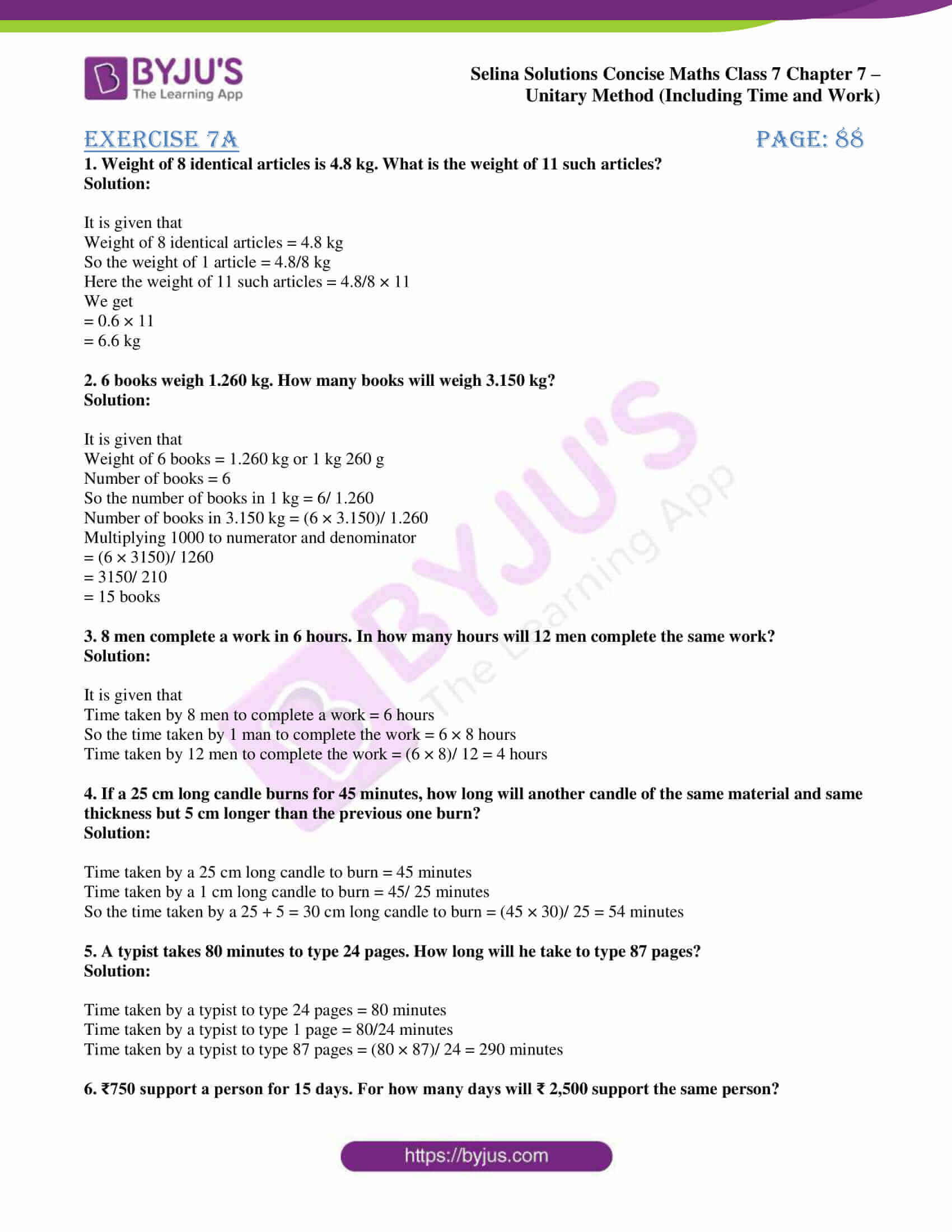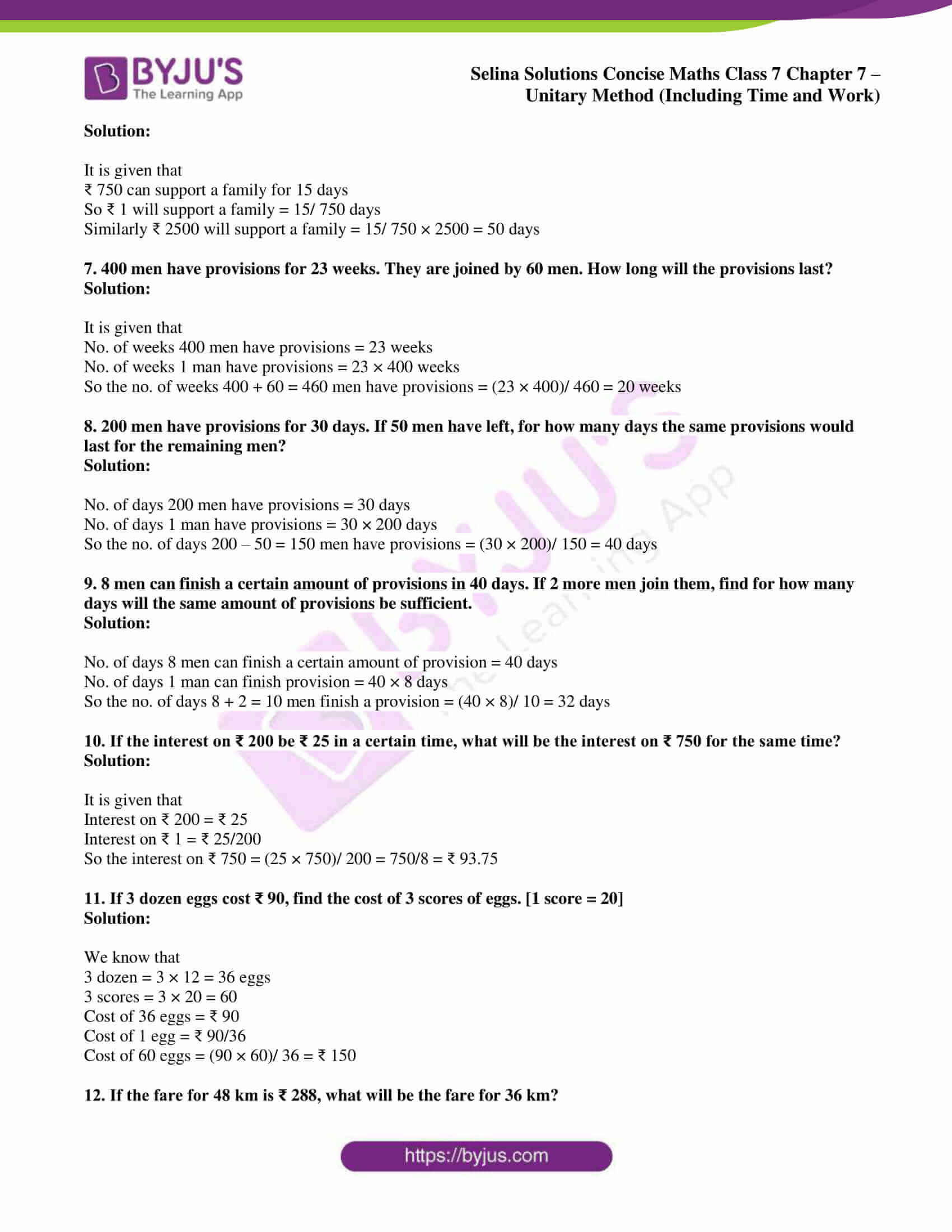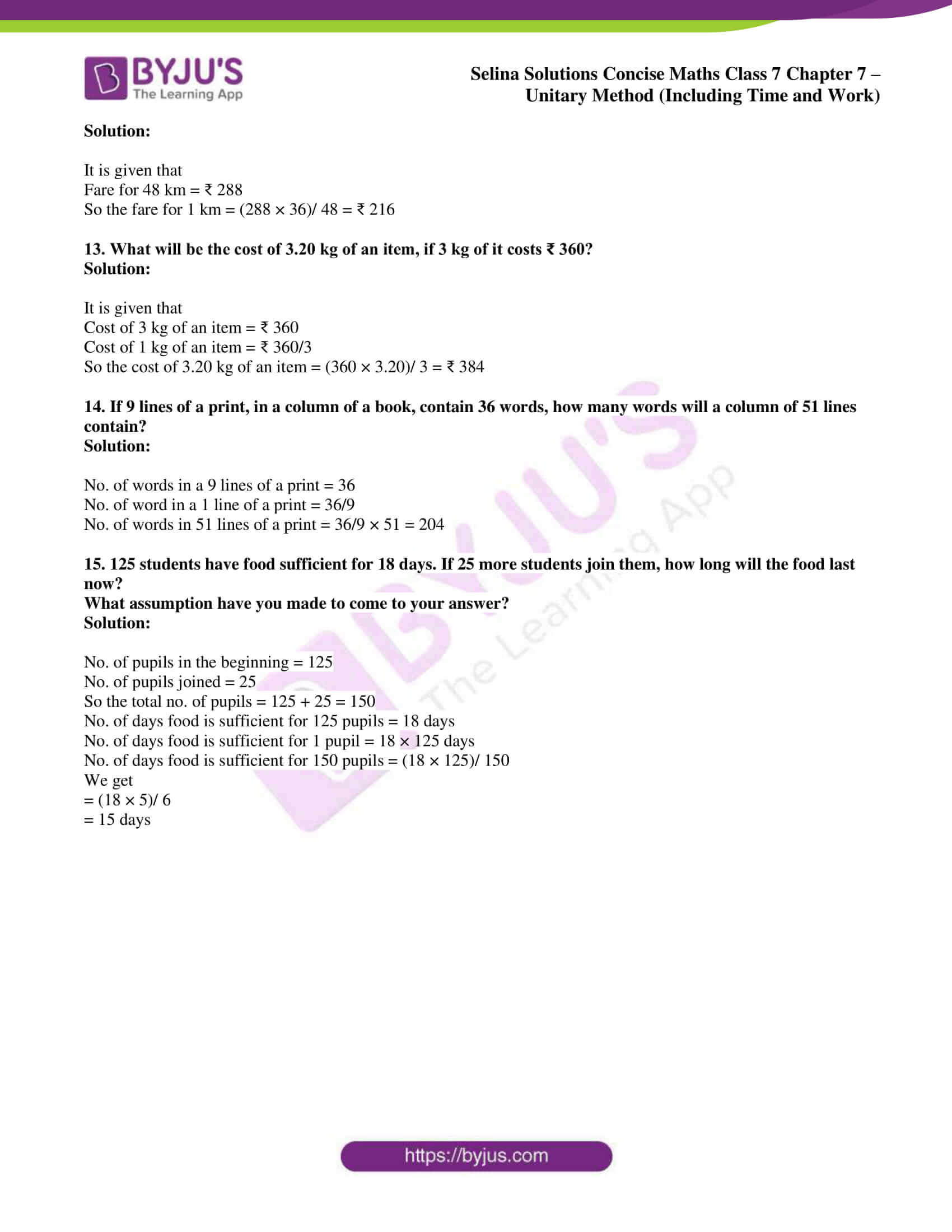# Selina Solutions Concise Maths Class 7 Chapter 7: Unitary Method (Including Time and Work) Exercise 7A

Selina Solutions Concise Maths Class 7 Chapter 7 Unitary Method (Including Time and Work) Exercise 7A has problems on direct and inverse variation, along with numerous examples. The process in which the value of one quantity is calculated and then of the given quantity is called the unitary method. Students who find difficulties in solving the exercise wise problems practise the textbook questions by referring to the solutions PDF. Here, Selina Solutions Concise Maths Class 7 Chapter 7 Unitary Method (Including Time and Work) Exercise 7A, PDF links are available with a free download option.

## Selina Solutions Concise Maths Class 7 Chapter 7: Unitary Method (Time and Work) Exercise 7A Download PDF### Access Selina Solutions Concise Maths Class 7 Chapter 7: Unitary Method (Time and Work) Exercise 7A

1. Weight of 8 identical articles is 4.8 kg. What is the weight of 11 such articles?

Solution:

It is given that

Weight of 8 identical articles = 4.8 kg

So the weight of 1 article = 4.8/8 kg

Here the weight of 11 such articles = 4.8/8 × 11

We get

= 0.6 × 11

= 6.6 kg

2. 6 books weigh 1.260 kg. How many books will weigh 3.150 kg?

Solution:

It is given that

Weight of 6 books = 1.260 kg or 1 kg 260 g

Number of books = 6

So the number of books in 1 kg = 6/ 1.260

Number of books in 3.150 kg = (6 × 3.150)/ 1.260

Multiplying 1000 to numerator and denominator

= (6 × 3150)/ 1260

= 3150/ 210

= 15 books

3. 8 men complete a work in 6 hours. In how many hours will 12 men complete the same work?

Solution:

It is given that

Time taken by 8 men to complete a work = 6 hours

So the time taken by 1 man to complete the work = 6 × 8 hours

Time taken by 12 men to complete the work = (6 × 8)/ 12 = 4 hours

4. If a 25 cm long candle burns for 45 minutes, how long will another candle of the same material and same thickness but 5 cm longer than the previous one burn?

Solution:

Time taken by a 25 cm long candle to burn = 45 minutes

Time taken by a 1 cm long candle to burn = 45/ 25 minutes

So the time taken by a 25 + 5 = 30 cm long candle to burn = (45 × 30)/ 25 = 54 minutes

5. A typist takes 80 minutes to type 24 pages. How long will he take to type 87 pages?

Solution:

Time taken by a typist to type 24 pages = 80 minutes

Time taken by a typist to type 1 page = 80/24 minutes

Time taken by a typist to type 87 pages = (80 × 87)/ 24 = 290 minutes

6. ₹750 support a person for 15 days. For how many days will ₹ 2,500 support the same person?

Solution:

It is given that

₹ 750 can support a family for 15 days

So ₹ 1 will support a family = 15/ 750 days

Similarly ₹ 2500 will support a family = 15/ 750 × 2500 = 50 days

7. 400 men have provisions for 23 weeks. They are joined by 60 men. How long will the provisions last?

Solution:

It is given that

No. of weeks 400 men have provisions = 23 weeks

No. of weeks 1 man have provisions = 23 × 400 weeks

So the no. of weeks 400 + 60 = 460 men have provisions = (23 × 400)/ 460 = 20 weeks

8. 200 men have provisions for 30 days. If 50 men have left, for how many days the same provisions would last for the remaining men?

Solution:

No. of days 200 men have provisions = 30 days

No. of days 1 man have provisions = 30 × 200 days

So the no. of days 200 – 50 = 150 men have provisions = (30 × 200)/ 150 = 40 days

9. 8 men can finish a certain amount of provisions in 40 days. If 2 more men join them, find for how many days will the same amount of provisions be sufficient.

Solution:

No. of days 8 men can finish a certain amount of provision = 40 days

No. of days 1 man can finish provision = 40 × 8 days

So the no. of days 8 + 2 = 10 men finish a provision = (40 × 8)/ 10 = 32 days

10. If the interest on ₹ 200 be ₹ 25 in a certain time, what will be the interest on ₹ 750 for the same time?

Solution:

It is given that

Interest on ₹ 200 = ₹ 25

Interest on ₹ 1 = ₹ 25/200

So the interest on ₹ 750 = (25 × 750)/ 200 = 750/8 = ₹ 93.75

11. If 3 dozen eggs cost ₹ 90, find the cost of 3 scores of eggs. [1 score = 20]

Solution:

We know that

3 dozen = 3 × 12 = 36 eggs

3 scores = 3 × 20 = 60

Cost of 36 eggs = ₹ 90

Cost of 1 egg = ₹ 90/36

Cost of 60 eggs = (90 × 60)/ 36 = ₹ 150

12. If the fare for 48 km is ₹ 288, what will be the fare for 36 km?

Solution:

It is given that

Fare for 48 km = ₹ 288

So the fare for 1 km = (288 × 36)/ 48 = ₹ 216

13. What will be the cost of 3.20 kg of an item, if 3 kg of it costs ₹ 360?

Solution:

It is given that

Cost of 3 kg of an item = ₹ 360

Cost of 1 kg of an item = ₹ 360/3

So the cost of 3.20 kg of an item = (360 × 3.20)/ 3 = ₹ 384

14. If 9 lines of a print, in a column of a book, contain 36 words, how many words will a column of 51 lines contain?

Solution:

No. of words in a 9 lines of a print = 36

No. of word in a 1 line of a print = 36/9

No. of words in 51 lines of a print = 36/9 × 51 = 204

15. 125 students have food sufficient for 18 days. If 25 more students join them, how long will the food last now?

Solution:

No. of pupils in the beginning = 125

No. of pupils joined = 25

So the total no. of pupils = 125 + 25 = 150

No. of days food is sufficient for 125 pupils = 18 days

No. of days food is sufficient for 1 pupil = 18 × 125 days

No. of days food is sufficient for 150 pupils = (18 × 125)/ 150

We get

= (18 × 5)/ 6

= 15 days